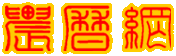回复帖子发帖表情--  作者：解味石头
--
excel:{  L0=-2318.19281=360+MOD(-2318.19281,360)= 201.80719;  }
js:    {  L0=-2318.19281=360+(-2318.19281%360)= 201.80719;      }

--  作者：zhanglei1953
--
Lo= -2318°.19281 = 201°.80719，实际上就是一个对360°求模的问题，VB里有相应语句MOD，如果不会用，可以采用笨办法，用-2318°.19281 多次加上360°，直到得到一个正数的角度，就是201°.80719。

--  作者：myyh
--

--  作者：myyh
--

Function jd(y As Integer, m As Integer, d As Double) As Double  '儒略日计算
'传统上儒略日的计数从格林尼治平午，即世界时12点开始
glg = y & Format(m, "00") & Format(Int(d), "00") & d - Int(d)
Dim a As Double, b As Double
If m <= 2 Then
y = y - 1: m = m + 12
End If
a = Int(y / 100)
b = 2 - a + Int(a / 4)
If glg <= 15821015.5 Then b = 0 '儒略日格利高历界限15821015 12:00:00pm日
jd = Int(365.25 * (y + 4716)) + Int(30.6001 * (m + 1)) + d + b - 1524.5
End Function

--  作者：myyh
--  [求助]Vb6求儒略日算法程序和一个问题

lo = 280.46645 + 36000.76983 * t + 0.0003032 * t ^ 2

Lo= -2318°.19281 = 201°.80719

Function jd(sdate As Date) As Double  '儒略日计算
Dim y As Integer, m As Integer, d As Double
Dim a As Double, b As Double, jd As Double
y = Year(sdate): m = Month(sdate): d = Day(sdate)
If m <= 2 Then
y = y - 1: m = m + 12
End If
a = Int(y / 100)
b = 2 - a + Int(a / 4)
If y <= 1582 Then b = 0    'And m <= 10 And d <= 15 Then b = 0'儒略日格利高历界限15821015日
jd = Int(365.25 * (y + 4716)) + Int(30.6001 * (m + 1)) + d + b - 1524.5
End Function

[此贴子已经被作者于2012-7-3 23:36:26编辑过]